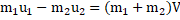# A body of mass 2 kg moving with a velocity 3 m/s collides with a body of mass 1 kg moving with a velocity of 4 m/s in opposite direction. Ifthe collision is head on and completely inelastic, then a) Both particles move together with velocity (2/3) m/s b) The momentum of system is 2 kg m/s throughout c) The momentum of system is 10 kg m/s d) The loss of KE of system is (49/3)J

## Question ID - 100823 :- A body of mass 2 kg moving with a velocity 3 m/s collides with a body of mass 1 kg moving with a velocity of 4 m/s in opposite direction. Ifthe collision is head on and completely inelastic, then a) Both particles move together with velocity (2/3) m/s b) The momentum of system is 2 kg m/s throughout c) The momentum of system is 10 kg m/s d) The loss of KE of system is (49/3)J

3537

 (a,b,d) In completely inelastic collision, bodies coalesceNet momentum of systemNext Question :

Figure gives force versus time graph. The force is acting on a particle of mass 2.0 kg at rest atand particle is moving in one dimension. Which of the following are correct?a) The impulse of the force in the time intervaltoisb) The velocity change in the intervaltoisc) The kinetic energy of the particle atisd) The kinetic energy of the particle atis